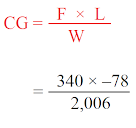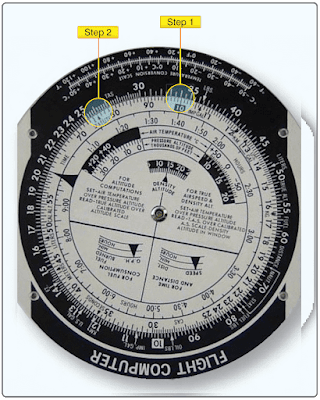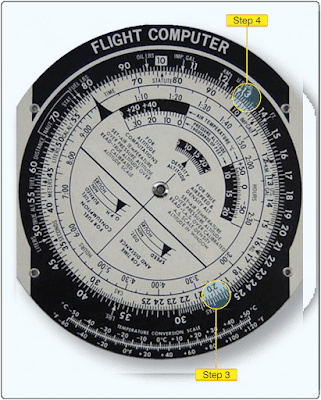# E6-B Flight Computer

The E6-B uses a special kind of slide rule. Instead of its scales going from 1 to 10, as on a normal slide rule, both scales go from 10 to 100. The E6-B cannot be used for addition or subtraction, but it is useful for making calculations involving multiplication and division. Its accuracy is limited, but it is sufficiently accurate for most weight and balance problems.

The same problem that was just solved with the electronic calculator can be solved on an E6-B by following these steps:First, multiply 340 by 78 (disregard the minus sign) [Figure 1]:Figure 1. E6-B computer set up to multiply 340 by 78

• Step 1- place 10 on the inner scale (this is the index opposite 34 on the outer scale that represents 340).

• Step 2- opposite 78 on the inner scale, read 26.5 on the outer scale.
Determine the value of these digits by estimating: 300 × 80 = 24,000, so 340 × 78 = 26,500.

Then, divide 26,500 by 2,006 [Figure 2].Figure 2. E6-B computer set up to divide 26,500 by 2,006

• Step 3- on the inner scale, place 20, which represents 2,006 opposite 26.5 on the outer scale (26.5 represents 26,500).

• Step 4- opposite the index, 10, on the inner scale read 13.2 on the outer scale.
Determine the value of 13.2 by estimating: 20,000 ÷ 2000 = 10, so 26,500 ÷ 2,006 = 13.2.
The arm (–78) is negative, so the CG is also negative.

The CG is –13.2 inches or 13.2 inches ahead of the datum.

RELATED POSTS